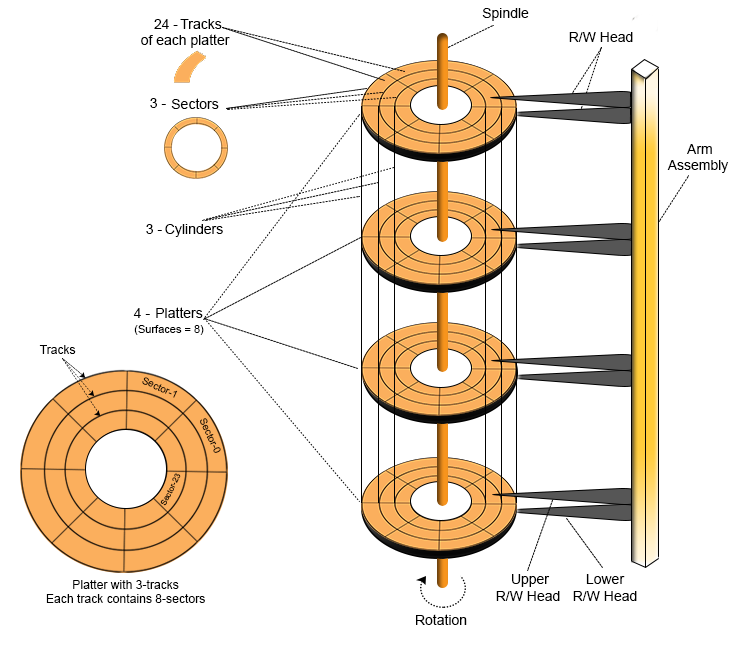Select Page

# Hard Disk Structure In OS

Hard disk is secondary storage which stores the large amount of data. The Hard disk drive contains a dozens of disks.  These disks are also known as platters. These platters are mount over the spindle which rotates in any direction i.e. clockwise or anti-clockwise. Lets look at hard disk structure in OS.## Platter

• The Platter is made of aluminum or iron oxide.
• Platter diameter range is 1.8 inches to 5.25 inches
• Each platter contains 2 surfaces and 2 Read/Write Head. One Read/Write head requires for one surface of the platter. So, other R/W head use for other surface to store the information’s.
• Every platter holds the same no. of tracks.
• Multiple platters increase the storage capacity.

• R/W Heads moves forth and back over the platter surfaces to Read or Write the data on sectors.
• Read/Write heads does not touch to platters surface. The data written over the platter surface is done through magnetic field. If R/W Head touches over the surface of platter then bad sectors may creates. Had disk may damage due to these bad sectors.

## Tracks

• Circular areas of disk are known as tracks.
• There may be more than a 1000 tracks on a 3.5 inch hard disk and sector size. Track Numbering start with zero at outermost track.

## Sectors

Tracks are further divided into number of small units; these small units are known as sectors. Sectors are the smallest physical storage units on disk. Size of each sector is almost always 512 Bytes. Sector Numbering start with 1 at outermost tracks.

## Cylinder

All Corresponding tracks with same radius of all platters in the Hard disk are known as cylinders.  In simple words we say

`“Each tack of all platters with same radius is called a cylinder”`

So, Number of tracks in platter is always equal to number of cylinders. For example, a hard disk, where each platter contains 600 tracks then the number of cylinders will also be 600 in the hard disk.

## Cluster

Cluster is also known as blocks. Group of sectors makes a cluster. There may be 64 or sectors in a cluster. These clusters are used by OS to Read/Write the data.

## Disk Capacity

As we know there are number of platters in the hard disk. Each platter contains two R/W heads. There are number of cylinder/tracks in the hard disk. Each track is divided into number of sectors. And each sector has some size but mostly size of sector is 512 Bytes.

Question 01: Let suppose there are 8 platters in hard disk drive. As each platters has two surfaces so 16 surfaces will be there in hard drive. Therefore, required R/W head will also be 16. Suppose, there are 1,024 cylinders and 128 sectors in each track. The sector size is 512 bytes.Then

```A). Calculate Disk Size?
B). How many number of bits required to represent the disk size?```

Part A. Solution

```Size of Hard Disk = Cylinder x Heads X Sectors x Sector-Size
= 1,024 x 16 x 128 x 512 Bytes
=  210 + 24 + 27 + 29 Bytes = 230Bytes = 2GB```

Part B. Solution

` As 2GB = 230 So, 30 bits are required to represent  2GB hard disk.`

Question-02:  Find the size of Single platter if track size 1KB and there are 1024 cylinder?

Solution: As there is a single platter So, Heads requirement will be 2.

```Size of Platter = Cylinder x Heads X track size (because, Track size = Sectors x Sector-Size)
= 210 + 21 + 210 Bytes
=21+220 Bytes = 2MB```

Question 03: Why does sector number addressing in CHS (Cylinder Head Sector) start at sector 1 and not 0?

Answer: For all translation modes, Cylinder (C) =0, Head (H) =0 and Sector (S) =1 is equivalent to Logical Block Address (LBA) =0. So the value of Sector is always 1.

## Logical structure

Logical division of hard disk is generally divided into 5 basic terms which are

• MBR (Master Boot Record)
• DBR (DOS Boot Record)
• FAT (File Allocation Tables)
• Root Directory
• Data Area

Help Other’s By Sharing…